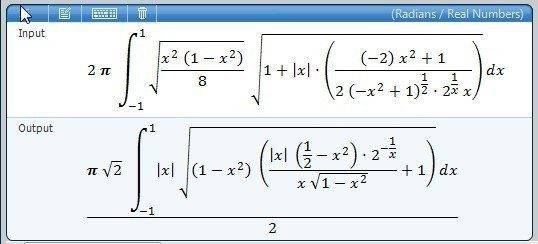# Surface of revolution

gimpycpu
Greeting everyone I am trying in integrate this function, to obtain the surface of revolution of a function.

1. Relevant equations

$\int_{-1}^{1} 2\pi\sqrt\frac{x^2 (1 - x^2)}{8}\sqrt{1+(\left| x\right | \frac{-2x^2+1}{2(-x^2+1)^{1/2}*2^{1/2}x}})^2$

2. The attempt at a solution

I tried to calculate the limit when x = 1 but I can't seems to get the result

If someone would just have a hint on how to calculate the limit of this integral I would appreciate.

Thank you

Jonathan

## Answers and Replies

ohms law
heh, I've been having troubles with integrating these as well.
The textbook just punts, so I've kinda given up trying to compute exact areas of these (even the solutions manual is setting the integrals up and then using a calculator/CAS to approximate a numerical value).

that's a crazy f(x) though. Are you sure that's what was given in the original problem?
Pull the $2\pi$ out to the front before trying to calculate it, though.

gimpycpu
Hehe I know right the original function is

$\sqrt{ \frac{x^2 (1 - x^2)}{8}}$

so I have to do the surface of revolution formula on this

which is defined

$S = \int_a^b2\pi f(x)\sqrt{1+(\frac{dy}{dx})^2}dx$

ohms law
Right... I assume that the derivative there is correct (although, it looks strange for some reason. Product/quotient rule derivatives often do though), and that it's been squared correctly (which is probably another reason why it looks wierd).

Anyway, here's what MS Math does with it:I'm not even going to try to punch all of that into my TI-84 (zOMG), especially since it would proably just choke on it anyway. I think that's as far as you can take that problem (without some additional calculus that I have no idea how to perform).

gimpycpu
I think that I found it,

you can reduce the big squareroot of death to a mere
$\sqrt{1+\frac{-1}{-∞}} = 1$ so the final equation is equal to

$\int_{-1}^{1} 2\pi\sqrt\frac{x^2 (1 - x^2)}{8} * 1 dx$
$= 2\pi\int_{-1}^{1} \sqrt\frac{x^2 (1 - x^2)}{8}dx$

which is a lot simplier to integrate

Last edited:
ohms law
huh... cool. How'd you do that simplification, though? It looks vaguely familiar for some reason (the -1/-infinity), but I can't place it.